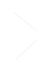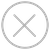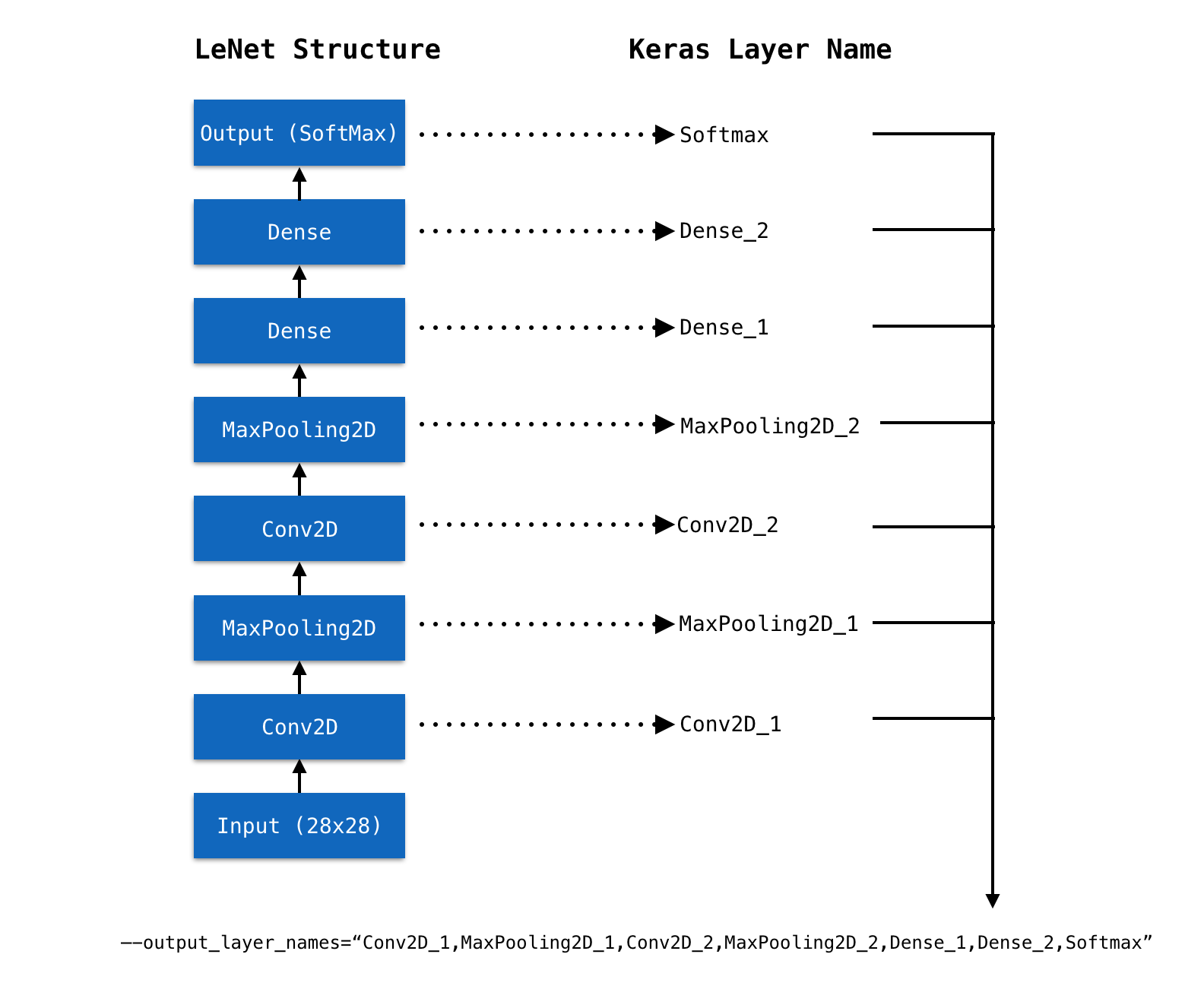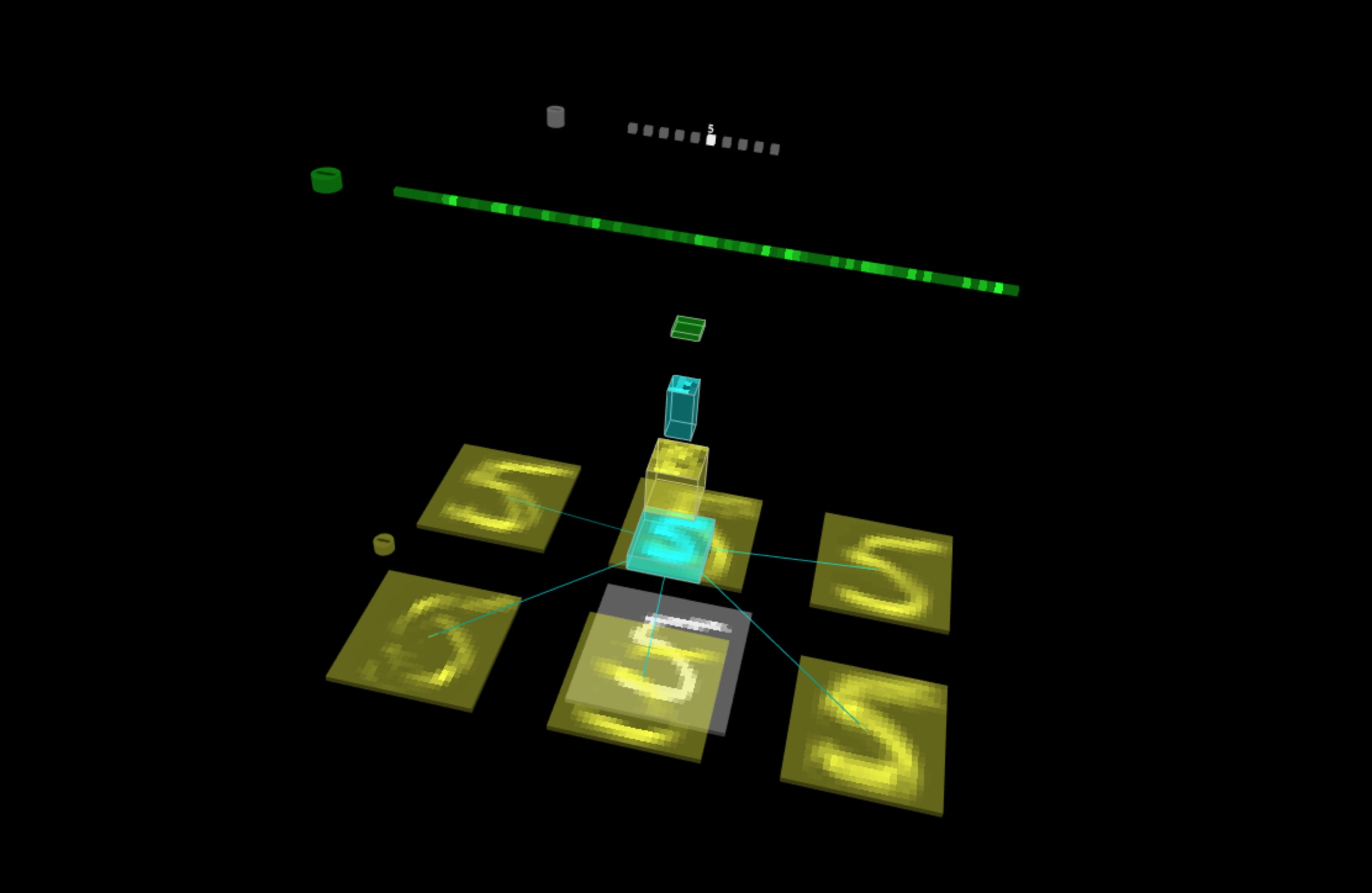TensorSpace.js
Getting StartBasic ConceptsTensorSpace ConverterModel PreprocessingModelsLayersMerge FunctionVisualize pre-trained Keras model using TensorSpace and TensorSpace-Converter
Introduction
In the following chapter, we will introduce the usage and workflow of visualizing Keras model using TensorSpace and TensorSpace-Converter. In this tutorial, we will convert Keras models with TensorSpace-Converter and visualize the converted models with TensorSpace.
This example uses LeNet trained with MNIST dataset. If you do not have any existed model in hands, you can use this script to train a LeNet TensorFlow.js model. We also provide pre-trained Keras LeNet models for this example.
Sample Files
The sample files that are used in this tutorial are listed below:
filter_center_focus pre-trained Keras models
Preprocess
First we will use TensorSpace-Converter to preprocess pre-trained different formats of Keras models.
Combined .h5
For a Keras model, topology and weights may be saved in a single HDF5 file, i.e. xxx.h5. Use the following convert script:
``````\$ tensorspacejs_converter \
--input_model_from="keras" \
--input_model_format="topology_weights_combined" \
--output_node_names="Conv2D_1,MaxPooling2D_1,Conv2D_2,MaxPooling2D_2,Dense_1,Dense_2,Softmax" \
./rawModel/combined/mnist.h5 \
./convertedModel/``````
wb_sunnyNote:
• filter_center_focus Set input_model_from to be keras.
• filter_center_focus Set input_model_format to be topology_weights_combined.
• filter_center_focus Set .h5 file's path to positional argument input_path.
• filter_center_focus Get out the Keras layer names of model, and set to output_layer_names like Fig. 1.
• filter_center_focus TensorSpace-Converter will generate preprocessed model into convertedModel folder, for tutorial propose, we have already generated a model which can be found in this folder.
Separated .json & .h5
For a Keras model, topology and weights may be saved in separated files, i.e. a topology file xxx.json and a weights file eee.h5. Use the following convert script:
``````\$ tensorspacejs_converter \
--input_model_from="keras" \
--input_model_format="topology_weights_separated" \
--output_node_names="Conv2D_1,MaxPooling2D_1,Conv2D_2,MaxPooling2D_2,Dense_1,Dense_2,Softmax" \
./rawModel/separated/topology.json,./rawModel/separated/weight.h5 \
./convertedModel/``````
wb_sunnyNote:
• filter_center_focus Set input_model_from to be keras.
• filter_center_focus Set input_model_format to be topology_weights_separated.
• filter_center_focus In this case, the model have two input files, merge two file's paths and separate them with comma (.json first, .h5 last), and then set the combined path to positional argument input_path.
• filter_center_focus Get out the Keras layer names of model, and set to output_layer_names like Fig. 1.
• filter_center_focus TensorSpace-Converter will generate preprocessed model into convertedModel folder, for tutorial propose, we have already generated a model which can be found in this folder.Fig. 1 - Set Keras layer names to output_layer_names
After converting, we shall have the following preprocessed model:Fig. 2 - Preprocessed Keras model
wb_sunnyNote:
• filter_center_focus There are two types of files created:
• flare One model.json file, describe the structure of our model (defined multiple outputs).
• flare Some weight files which contains trained weights. The number of weight files is dependent on the size and structure of the given model.
Then Apply TensorSpace API to construct visualization model.
``````let model = new TSP.models.Sequential( modelContainer );

``````model.load( {